# Temperature and Heat Temperature Scales Celcius Farenheit Kelvin

• Slides: 25Temperature and HeatTemperature Scales Celcius Farenheit Kelvin 212 100 373. 15 32 0 273. 15 Water boils Water freezes NOTE: K=0 is “absolute zero”, meaning (almost) zero KE/molecule 14Temp Scales Question l Two cups of coffee are heated to 100 degrees Fahrenheit. Cup 1 is then heated an additional 20 degrees Centigrade, cup 2 is heated an additional 20 Kelvin. Which cup of coffee is hotter? A) One B) Two C) Same 20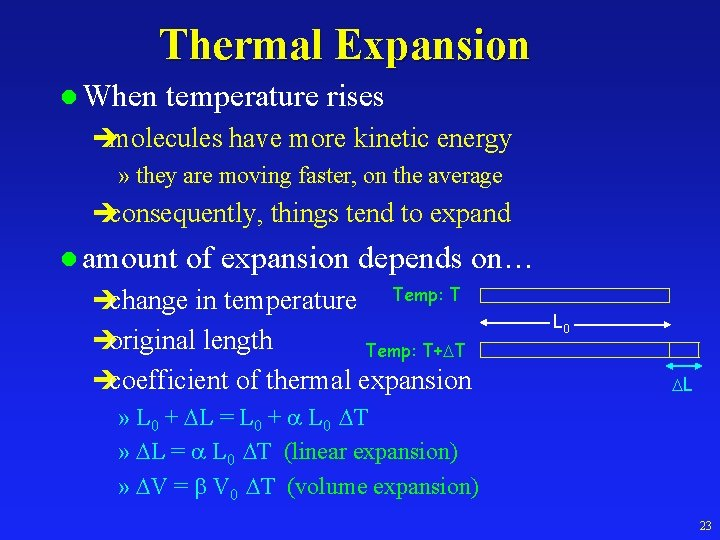Thermal Expansion l When temperature rises èmolecules have more kinetic energy » they are moving faster, on the average èconsequently, things tend to expand l amount of expansion depends on… èchange in temperature èoriginal length Temp: T L 0 Temp: T+ T ècoefficient of thermal expansion » L 0 + L = L 0 + L 0 T » L = L 0 T (linear expansion) » V = V 0 T (volume expansion) L 23Density Question As you heat a block of aluminum from 0 C to 100 C its density A. Increases B. Decreases T = 100 C C. Stays the same T=0 C M, V 0 r 0 = M / V 0 M, V 100 r 100 = M / V 100 < r 0 26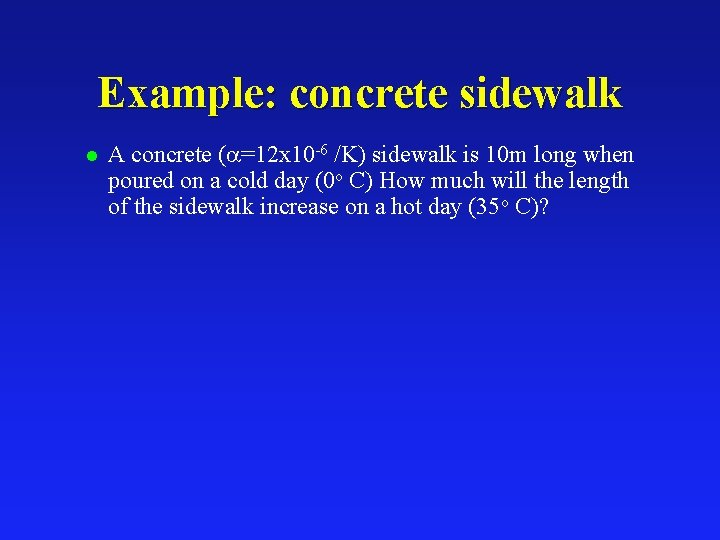Example: concrete sidewalk l A concrete ( =12 x 10 -6 /K) sidewalk is 10 m long when poured on a cold day (0 o C) How much will the length of the sidewalk increase on a hot day (35 o C)?Example: gas tank l A steel (β=36 x 10 -6 /K) gas tank has a volume of 75 lit. You fill it up with gasoline (β=950 x 10 -6 /K) early in the morning when it is cool During the day the temperature increases by 20 o C. How much gas will spill out?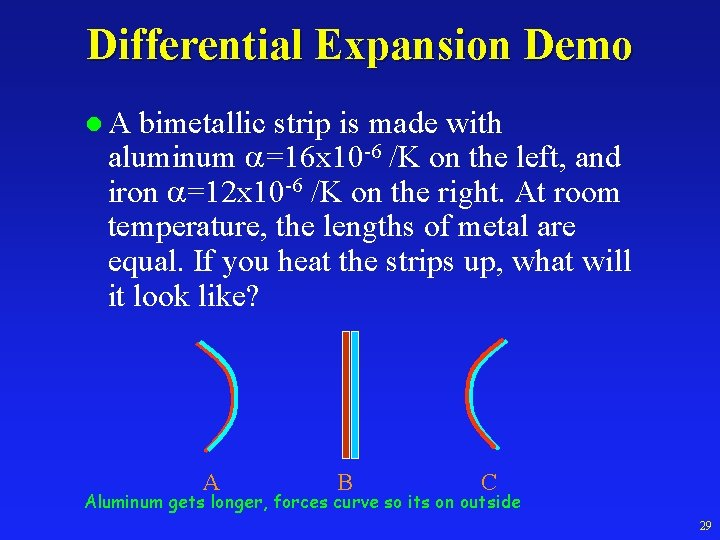Differential Expansion Demo l. A bimetallic strip is made with aluminum =16 x 10 -6 /K on the left, and iron =12 x 10 -6 /K on the right. At room temperature, the lengths of metal are equal. If you heat the strips up, what will it look like? A B C Aluminum gets longer, forces curve so its on outside 29Amazing Water l Water is very unusual in that it has a maximum density at 4 degrees C. That is why ice floats, and we exist! 30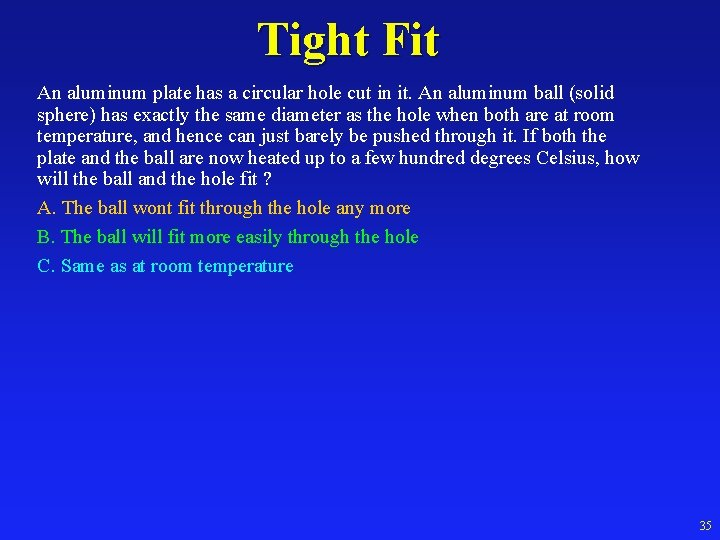Tight Fit An aluminum plate has a circular hole cut in it. An aluminum ball (solid sphere) has exactly the same diameter as the hole when both are at room temperature, and hence can just barely be pushed through it. If both the plate and the ball are now heated up to a few hundred degrees Celsius, how will the ball and the hole fit ? A. The ball wont fit through the hole any more B. The ball will fit more easily through the hole C. Same as at room temperature 35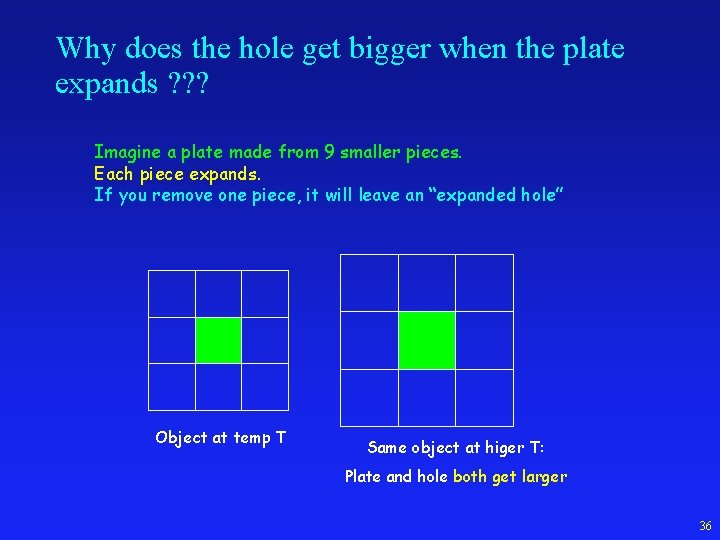Why does the hole get bigger when the plate expands ? ? ? Imagine a plate made from 9 smaller pieces. Each piece expands. If you remove one piece, it will leave an “expanded hole” Object at temp T Same object at higer T: Plate and hole both get larger 36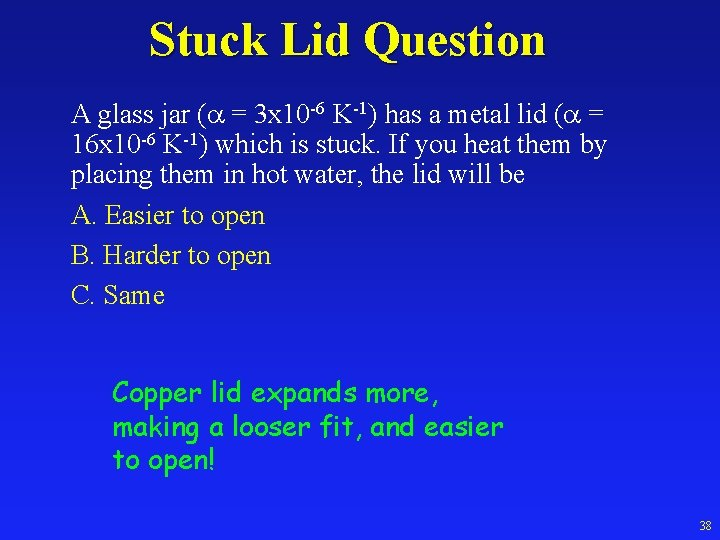Stuck Lid Question A glass jar ( = 3 x 10 -6 K-1) has a metal lid ( = 16 x 10 -6 K-1) which is stuck. If you heat them by placing them in hot water, the lid will be A. Easier to open B. Harder to open C. Same Copper lid expands more, making a looser fit, and easier to open! 38Heat l Definition: Flow of energy between two objects due to difference in temperature èNote: similar to WORK èObject does not “have” heat (it has energy: temp) l Units: Joule è 4186 Joules = 1 Calorie = 1000 calories = ècalorie: Amount of heat needed to raise 1 g of water 1ºC 10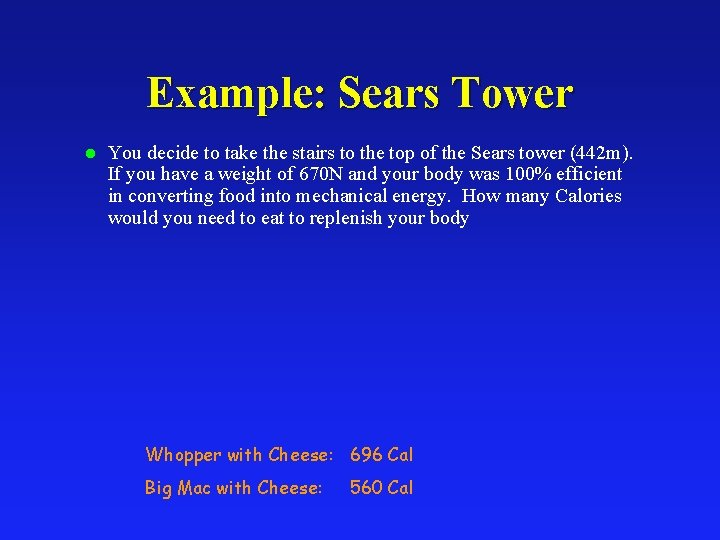Example: Sears Tower l You decide to take the stairs to the top of the Sears tower (442 m). If you have a weight of 670 N and your body was 100% efficient in converting food into mechanical energy. How many Calories would you need to eat to replenish your body Whopper with Cheese: 696 Cal Big Mac with Cheese: 560 CalSpecific Heat l Heat adds energy to object/system l IF system does NO work then: èHeat increases internal energy. Q = U èHeat increases temperature! l. Q = c m T èHeat required to increase Temp depends on amount of material (m) and type of material (c) l Q = cm T : “Cause” = “inertia” x “effect” (just like F=ma) è cause = Q è effect = T è inertia = cm (mass x specific heat capacity) l T = Q/cm (just like a = F/m) 15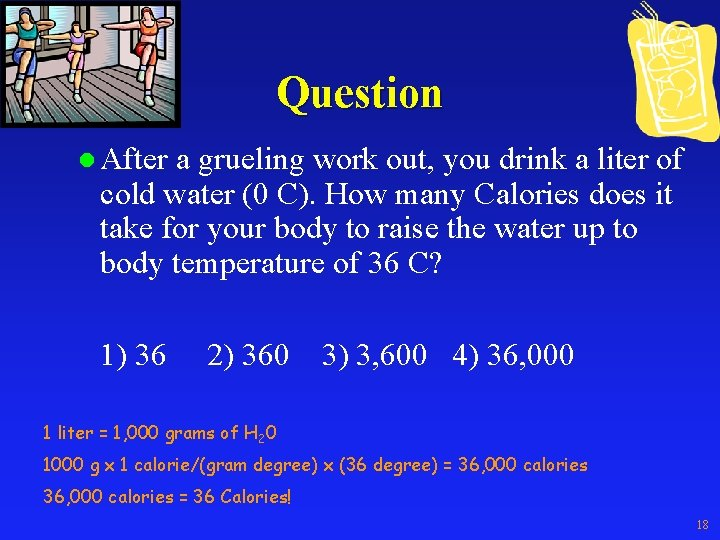Question l After a grueling work out, you drink a liter of cold water (0 C). How many Calories does it take for your body to raise the water up to body temperature of 36 C? 1) 36 2) 360 3) 3, 600 4) 36, 000 1 liter = 1, 000 grams of H 20 1000 g x 1 calorie/(gram degree) x (36 degree) = 36, 000 calories = 36 Calories! 18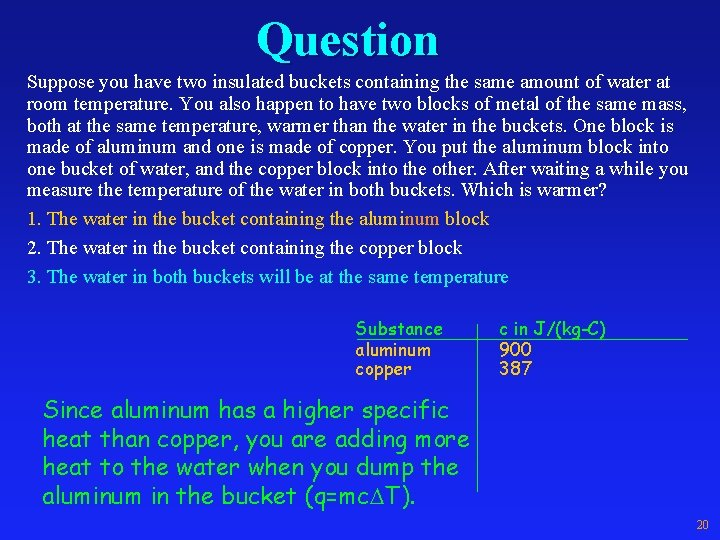Question Suppose you have two insulated buckets containing the same amount of water at room temperature. You also happen to have two blocks of metal of the same mass, both at the same temperature, warmer than the water in the buckets. One block is made of aluminum and one is made of copper. You put the aluminum block into one bucket of water, and the copper block into the other. After waiting a while you measure the temperature of the water in both buckets. Which is warmer? 1. The water in the bucket containing the aluminum block 2. The water in the bucket containing the copper block 3. The water in both buckets will be at the same temperature Substance aluminum copper c in J/(kg-C) 900 387 Since aluminum has a higher specific heat than copper, you are adding more heat to the water when you dump the aluminum in the bucket (q=mc T). 20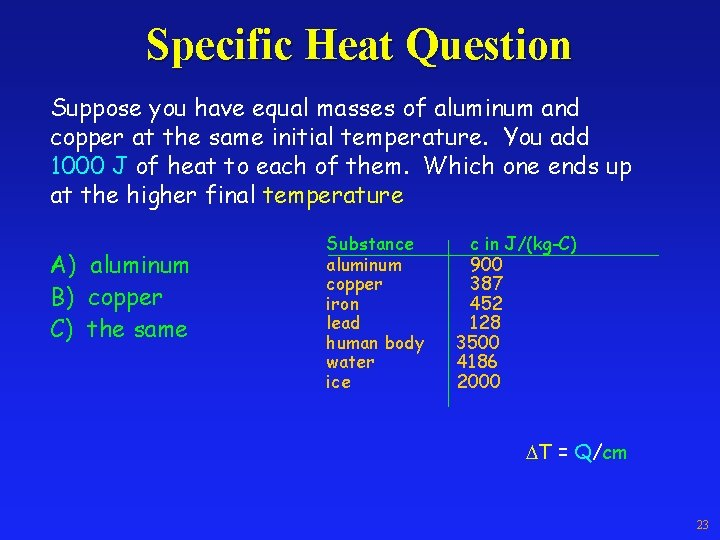Specific Heat Question Suppose you have equal masses of aluminum and copper at the same initial temperature. You add 1000 J of heat to each of them. Which one ends up at the higher final temperature A) aluminum B) copper C) the same Substance aluminum copper iron lead human body water ice c in J/(kg-C) 900 387 452 128 3500 4186 2000 T = Q/cm 23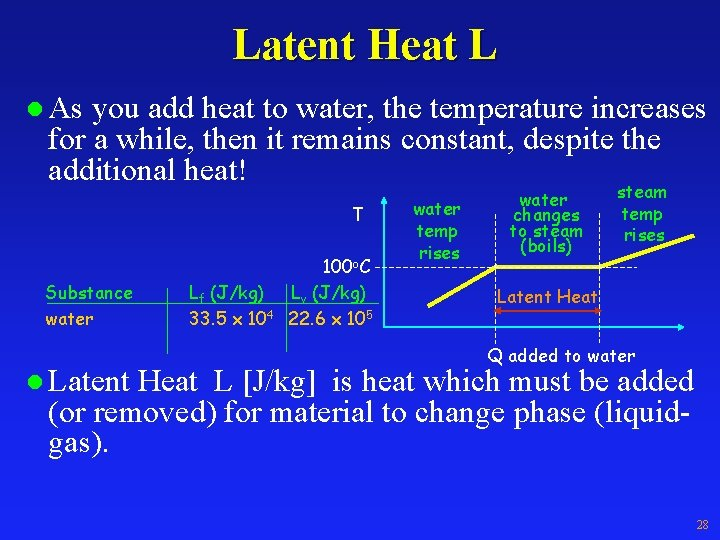Latent Heat L l As you add heat to water, the temperature increases for a while, then it remains constant, despite the additional heat! T Substance water l Latent Lf (J/kg) 33. 5 x 104 100 o. C Lv (J/kg) 22. 6 x 105 water temp rises water changes to steam (boils) steam temp rises Latent Heat Q added to water Heat L [J/kg] is heat which must be added (or removed) for material to change phase (liquidgas). 28Ice Question l Which will do a better job cooling your soda, a “cooler” filled with water at 0 C, or a cooler filled with ice at 0 C. A) Water B) About Same C) Ice Latent Heat L [J/kg] is heat which must be added (or removed) for material to change phase (liquidwater ice gas). ice T Substance water Lf (J/kg) 33. 5 x 104 0 o. C Lv (J/kg) 22. 6 x 105 temp rises changes to water (melts) temp rises Latent Heat Q added to water 30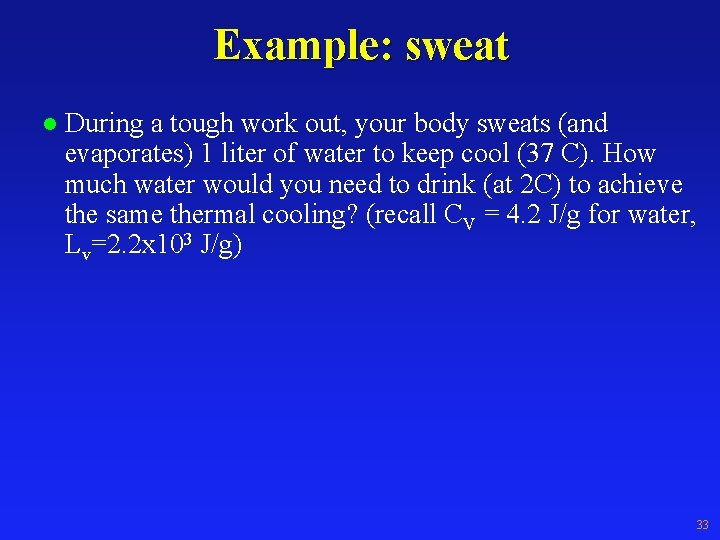Example: sweat l During a tough work out, your body sweats (and evaporates) 1 liter of water to keep cool (37 C). How much water would you need to drink (at 2 C) to achieve the same thermal cooling? (recall CV = 4. 2 J/g for water, Lv=2. 2 x 103 J/g) 33Question Summers in Phoenix Arizona are very hot (125 F is not uncommon), and very dry. If you hop into an outdoor swimming pool on a summer day in Phoenix, you will probably find that the water is too warm to be very refreshing. However, when you get out of the pool and let the sun dry you off, you find that you are quite cold for a few minutes (yes. . . you will have goose-bumps on a day when the air temperature is over 120 degrees). How can you explain this? 35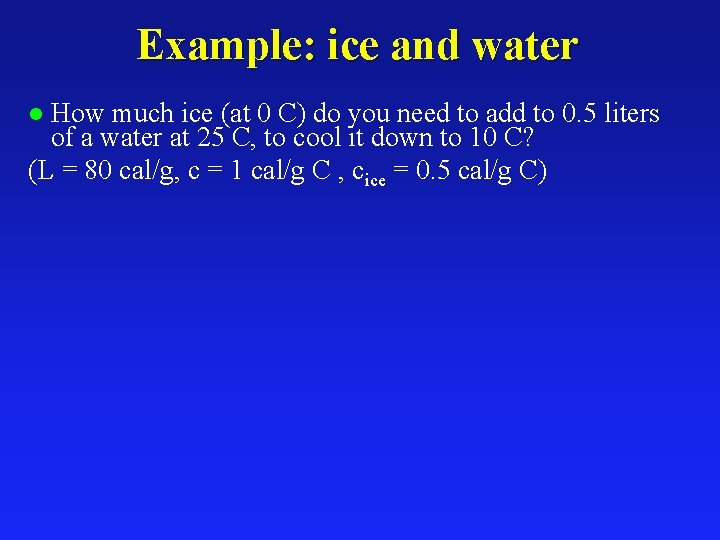Example: ice and water How much ice (at 0 C) do you need to add to 0. 5 liters of a water at 25 C, to cool it down to 10 C? (L = 80 cal/g, c = 1 cal/g C , cice = 0. 5 cal/g C) l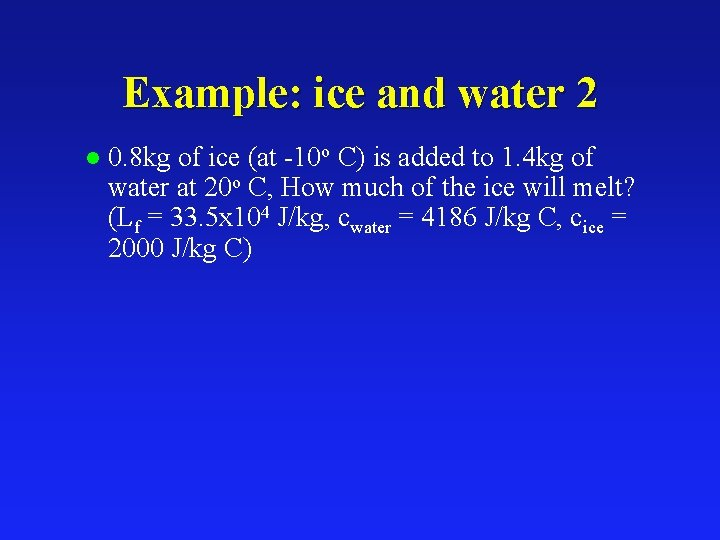Example: ice and water 2 l 0. 8 kg of ice (at -10 o C) is added to 1. 4 kg of water at 20 o C, How much of the ice will melt? (Lf = 33. 5 x 104 J/kg, cwater = 4186 J/kg C, cice = 2000 J/kg C)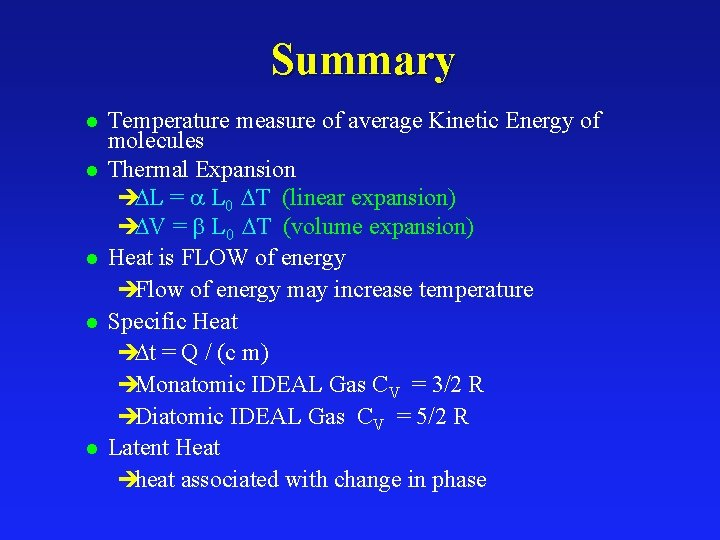Summary l l l Temperature measure of average Kinetic Energy of molecules Thermal Expansion è L = L 0 T (linear expansion) è V = L 0 T (volume expansion) Heat is FLOW of energy èFlow of energy may increase temperature Specific Heat è t = Q / (c m) èMonatomic IDEAL Gas CV = 3/2 R èDiatomic IDEAL Gas CV = 5/2 R Latent Heat èheat associated with change in phase